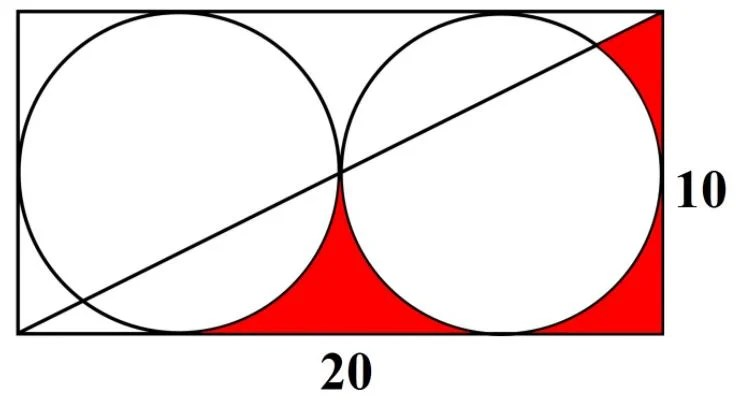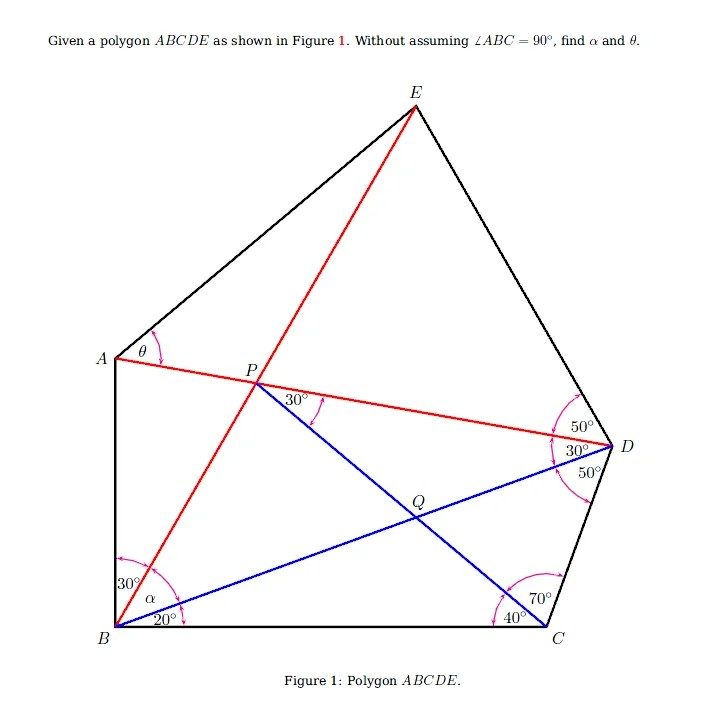# Brain Gym

Below are just some of the questions that I found interesting and ones that can give a good workout for our brain.

## Mathematics

Question 1

Jess wants 200 ribbons of length 110 cm for a party. However, the ribbons were sold at 25 m per tape. How many tapes will Jess need?
[Answer is 10. Hint: In real life, incomplete length ribbon is not usable.]

Question 2

Find the total area of the red regions in the diagram below.[Hint: you might need to find area of minor segment + alternate angles to find area of sector]

Question 3Answer is α = 40 degrees and θ = 50 degrees.

[Hint: Use sine rule and try multiplying the ratio to cancel the sides out to solve for angles.]

#### Extra resources

1. http://www.cambridgebrainsciences.com